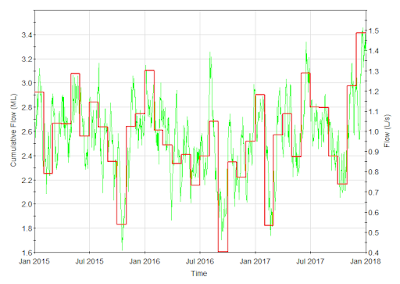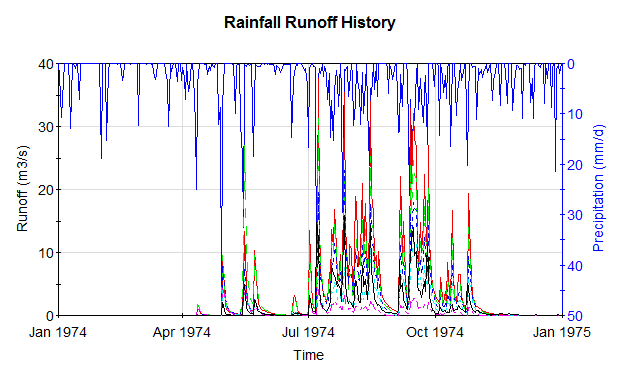## Monday, June 13, 2016

### Using Arrays in GoldSim

Posted by Ryan RoperMonthly cumulative flow results (see webinar 'Introduction to Arrays')
One of the most powerful features of GoldSim is its support for vector and matrix data types, or arrays. Whether you are modeling different age cohorts in a population dynamics model or you need to calculate monthly and/or yearly flow totals in a water resource model, the use of arrays can reduce complexity in your model and help you calculate useful results. If you are developing a contaminant transport model, using arrays is unavoidable since species properties are stored in vectors.

Proficiency with arrays in GoldSim is essential for many applications. Gaining a rudimentary understanding will require that you at least know how to create and use array label sets (named and indexed) and how to reference values in an array. I would definitely encourage you, though, to go beyond a basic proficiency and take advantage of the advanced capabilities that GoldSim provides. This blog post will point you to resources to help you get familiar with and start using arrays in GoldSim. I will highlight some example applications that range from very basic usage of arrays to sophisticated applications involving the use of array constructors.

GoldSim Help and Example Model

To get a basic understanding of using arrays in GoldSim you should go to Help Topics in GoldSim, under the Help menu, and browse through the ‘Using Vectors and Matrices’ section. You can find this by clicking the ‘Contents’ tab and expanding the ‘Advanced Modeling Concepts’ section. Of particular importance, whether you need only a basic proficiency with arrays or you want to use arrays in more advanced applications, is to know how to use array constructors. Be sure to look at the article ‘Defining Arrays in an Input Field Using Array Constructor Functions’. Also, take a look at the Arrays.gsm example model that comes with GoldSim. You can find this by going to Help|Example Models in GoldSim and looking in the ‘General Examples’ folder.

Introduction to Arrays Webinar

In November 2015, I did a webinar on using arrays. This webinar can be found here: Introduction to Arrays (you can also see it on YouTube here). Be sure to download the webinar materials, which includes PowerPoint slides and a number of example models. Topics covered in the webinar include the following: using array label sets, referencing array subitems, using arrays in expressions, using built-in array functions and using array constructors. The topic of array constructors was presented within the context of a hydrology application where yearly summary statistics on monthly cumulative flow amounts were calculated using array constructors and built-in array functions.

GoldSim Model Library

Finally, the GoldSim Model Library is an excellent resource to see many different ways that arrays can be used in GoldSim. Examples range from basic to advanced. Here are some basic models to look at:

Vector and Matrix Constructors: This model demonstrates the various ways in which array constructors can be used to construct vectors or matrices from different inputs.

Matrix/Vector Conversions: This model illustrates how to load values from a matrix into a vector and from a vector into a matrix using vector and matrix constructors. A target vector can be loaded from a matrix either by row or by column and likewise a target matrix can be loaded by row or column from a vector.

For more advanced examples, take a look at the following:

Monthly Cumulative Flows: This model shows how a vector constructor can be used to take a scalar time-varying input rate and calculate the accumulation over successive time periods (e.g. months). This is illustrated with a simple example where the input rate is 1 1/day. The result is a vector containing the number of days in each of the 12 months. In a subcontainer of the model, there is an example application of this strategy where accumulated volumes are calculated from a random flow rate input over 12 months.Calculation of flow accumulation during the month of October

Monthly and Annual Summaries: This model uses the same strategy as illustrated in the example above to calculate cumulative monthly volumes over 5 years. The results are stored in a matrix where the rows correspond to years and the columns correspond to months. With the results stored in this format, it is simple to calculate other quantities such as yearly totals or average monthly totals using GoldSim's built-in array functions (e.g. meanr(), meanc(), sumr(), sumc(), etc.).

Australian Water Balance Model (AWBM): This is a rainfall runoff model that uses a vector Reservoir element to model a single catchment.Schematic for Australian Water Balance Model (AWBM) for rainfall runoff

See also Australian Water Balance Model (Vectors), which models multiple subcatchments using a matrix Reservoir element.Australian Water Balance Model (AWBM) results with multiple catchments

For many more examples of using vectors and matrices in GoldSim, visit this page.